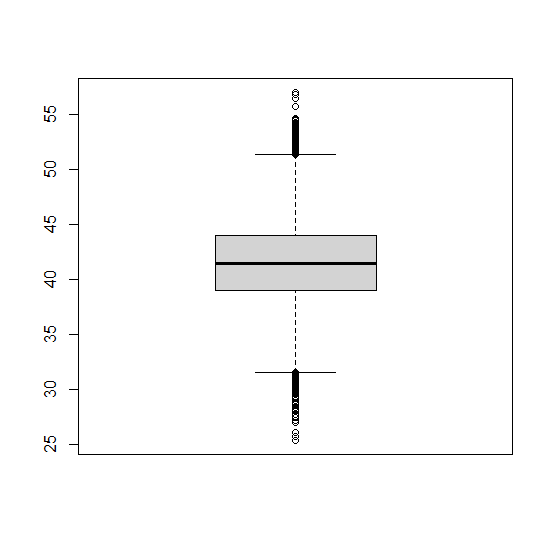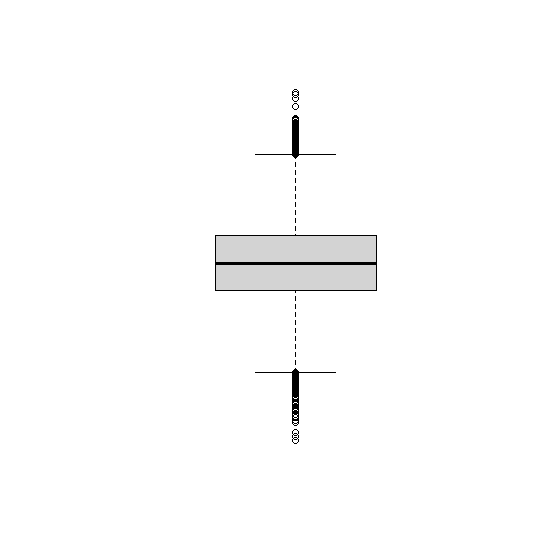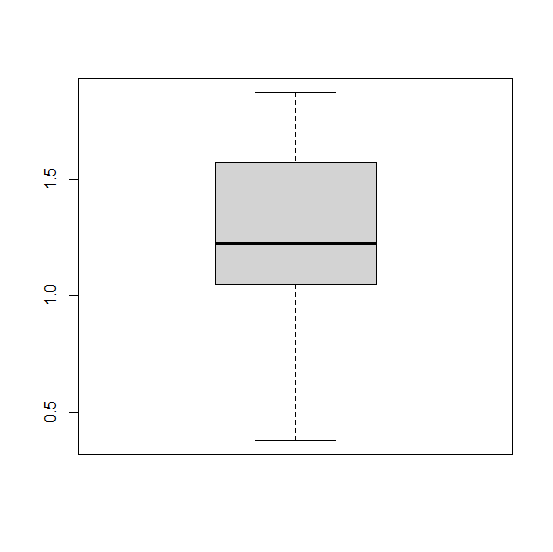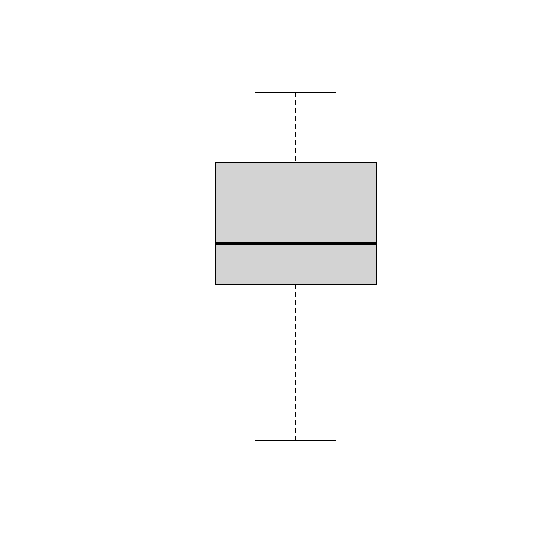# How to create boxplot in base R without axes labels?

The boxplot can be created by using boxplot function in base R but the Y−axis labels are generated based on the vector we pass through the function. If we want to remove the axis labels then axes = FALSE argument can be used. For example, if we have a vector x then the boxplot for x without axes labels can be created by using boxplot(x,axes=FALSE).

## Example

Live Demo

Consider the below vector x and creating boxplot −

set.seed(777)
x<−rnorm(50000,41.5,3.7)
boxplot(x)

## OutputCreating the boxplot without Y−axis labels −

boxplot(x,axes=FALSE)

## OutputLet’s have a look at another example −

## Example

Live Demo

y<−rnorm(10,1,0.5)
boxplot(y)

## OutputRemoving Y−axis labels −

boxplot(y,axes=FALSE)

## OutputWe can do the same for multiple boxplots if the data is contained in a data frame.